# Class 10 Science Chapter 12 Electricity

NCERT Solutions For Class 10 Science Chapter 12 Electricity. Question Answer.

Question 1.

What does an electric circuit mean?
A continuous and closed path of an electric current is called an electric circuit.

Question 2.
Define the unit of current.
One ampere is the current flowing through a circuit, when a charge of one coulomb flows through it in one second.

Question 3.
Calculate the number of electrons constituting one coulomb of charge.
Total charge, Q = n x e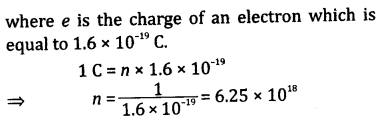Question 4.
Name a device that helps to maintain a potential difference across a conductor?
With the help of a cell or a battery.

Question 5.
What is meant by saying that potential difference between two points is 1 volt?
It means that 1 joule work is done in moving 1 coulomb of positive charge from one point to the other in an elecfric field.

Question 6.
How much energy is given to each coulomb of charge passing through a 6 V battery?
Potential difference (V) = 6 V
Charge (Q) = 1 coulomb
Energy = Q x V x 6 = 6 J

Question 7.
On what factors does the resistance of a conductor depend?
Resistance of a conductor depends on its material, length and area of cross-section.

Question 8.
Let the resistance of an electrical component remains constant while the potential difference across the two ends of the component decreases to half of its former value. What change will occur in the current through it?
According to Ohm’s law, we have:
I ∝ V or V ∝ I
Since the potential difference (V) decreases to half, i.e.
∴  V 2 I e
Hence, the current flowing through the component will become half.

Question 9.
Why are coils of electric toasters and electric irons made of an alloy rather than a pure metal?
This is because of the high resistivity and high melting point of the alloy. Also, they do not oxidise when red hot.

Question 10.
Draw a schematic diagram of a circuit consisting of a battery of three cells of 2 V each, a 5 Ω resistor, an 8 Ω resistor, and a 12 Ω resistor, and a plug key, all connected in series.Question 11.
Will current flow more easily through a thick wire or a thin wire of the same material when connected to the same source? Why?
The current will flow more easily through a wire which have comparatively less resistance. According to the relation  R 1 A , the thin wire has less resistance. So, the current will flow more easily through thin wire.

Question 12.
Judge the equivalent resistance when the following are connected in parallel:
(a) 1 Ω and 10 6 Ω
(b) 1 Ω and 10 3 Ω, and 10 6 Ω
(a) Approximately but less than 1 Ω, because in a parallel combination of resistors, the equivalent resistance is less than the least resistance.
(b) Same as in previous part of the question.

Question 13.
Redraw the circuit of previous question putting in an ammeter to measure the current through the resistors and a voltmeter to measure the potential difference across the 12 Ω resistor. What would be the readings in the ammeter and the voltmeter?
Total resistance, Rs = 5 + 8 + 12 = 25 Ω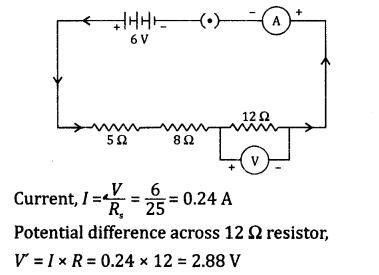Question 14.
An electric lamp of resistance 100 12, a toaster of resistance 50 11 and a water filter of resistance 500 12 are connected in parallel to a 220 V source. What is the resistance of an electric iron connected to the same source that takes as much current as all three appliances, and what is the current through it?
Given: Resistance of electric lamp, R 1 = 100Ω
Resistance of toaster, R 2 = 50Ω
Resistance of water filter, R 3 = 500Ω
Voltage, V = 220 V
Let R p be the resistance of electric iron. Then: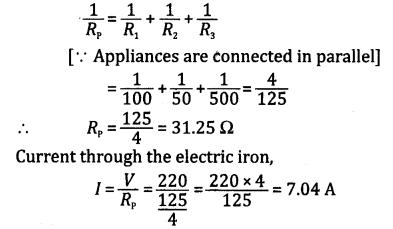Question 15.
What is  (a) the highest,  (b) the lowest total resistance that can be secured by combinations of four coils of resistances 4 Ω, 8 Ω, 12 Ω, 24 Ω?
(a) The highest resistance can be secured by connecting all the four coils in series.
∴ Equivalent resistance of series combination, R s= 4 + 8 + 12+ 24 = 48 Ω
Hence, the highest resistance that can be secured by given coils is 48 Ω.

(b) The lowest resistance can be secured by connecting all the four coils in parallel.
∴ Equivalent resistance of parallel combination,
1 R p = 1 4 + 1 8 + 1 12 + 1 24 = 1 2
or R p = 2 Ω
Hence, the lowest resistance that can be secured by given coils is 2 Ω.

Question 16.
Why does the cord of an electric heater not glow while the heating element does?
The resistance of metal used in cord has much lower than that its element. So the heat produced by the element is much more than that produced by the cord while passing the current.

Question 17.
Compute the heat generated while transferring 96000 coulomb of charge in one hour through a potential difference of 50 V.
Given, Q = 96000 C, V= 50 volt,
t = 1 hour = 60 x 60 = 3600 s
∴ Heat generated, H = VIt
= VQ = 50 x 96000
= 4800000 = 4.8 x 10 J

Question 18.
An electric iron of resistance 20 12 takes a current of 5 A. Calculate the heat developed in 30 s.
Heat developed is equal to the work done (W).
Given: R = 20 Ω, I = 5 A and t = 30 s
∴ W = I 2Rt = (5) 2 x 20 x 30
= 25 x 20 x 30 = 15,000 J
Hence, the heat developed by electric iron is 15,000 J.

Question 19.
What determines the rate at which energy is delivered by a current?
Electric power determines the rate at which energy is delivered by a current.

Question 20.
An electric motor takes 5 A from a 220 V line. Determine the power of the motor and the energy consumed in 2 hr.
Given: Current, I = 5 A, Voltage, V = 220 V and t= 2 h
Electric power, P=VI = 220 x 5 = 1100 W
∴ Energy consumed = Pt = 1100 x 7200
= 7920000 = 792 x 10 J

Question 21.
What are the advantages of connecting devices in parallel with the battery instead of connecting them in series?
(a) When electrical devices are connected in parallel, each is operated at the same voltage and if one of them goes off, the others are not affected. But when electrical devices are connected in series, this does not happen because the circuit is broken when any one of the devices fails.
(b) In parallel connection, the current divides itself through different devices. This is helpful when each device has different resistance and requires different current to operate. But in series connection this does not happen because the same current flows through all the devices.

Question 22.
How can three resistors of resistances 2 Ω, 3 Ω and 6 Ω be connected to give a total resistance of  (a) 4 Ω and
(b)
1 Ω ?
(a) In order to get a resistance of 4 Ω, we can connect resistors of 3 Ω and 6 Ω in parallel and this parallel combination in series with 2 Ω resistor.(b) In order to get 1 Ω resistance, we can connect all the three resistors in parallel.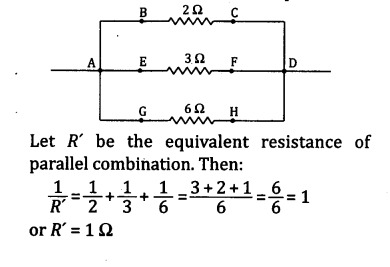Chapter End Questions

Question 1.
A piece of wire of resistance R is cut into five equal parts. These parts are then connected in parallel. If the equivalent resistance of this combination is R’, then the ratio  R R  is:
(a)  1 25
(b)  1 5
(c) 5
(d) 25
(d) 25

Question 2.
Which of the following terms does not represent the electrical power in a circuit?
(a) I 2R
(b) IR 2
(c) VI
(d)  V 2 R
(b) IR 2

Question 3.
Two conducting wires of the same material and of equal lengths and equal diameters are first ‘ connected in series and then parallel in a circuit across the same potential difference. The ratio of heat produced in series and parallel combination would be:
(a) 1 : 2
(b) 2 : 1
(c) 1 : 4
(d) 4 : 1
(c) 1 : 4

Question 4.
An electric bulb is rated 220 V and 100 W. When it is operated on 110 V, the )aower consumed will be
(a) 100 W ,
(b) 75 W
(c) 50 W
(d) 25 W
(d) 25 W

Question 5.
How is a voltmeter connected in the circuit to measure the potential difference between two points?
A voltmeter is connected in parallel to the circuit.

Question 6.
A copper wire has diameter 0.5 mm and resistivity of 1.6 x 10 8 Ω m. What will be the length of this wire to make its resistance 10 Ω? How much does the resistance change, if the diameter is doubled?
Given: Radius, r = 0.25 mm = 0.25 x 10 -3 m
Length of the wire, l = 1
Resistance, R =10 ΩQuestion 7.
The valued of current I flowing in a given resistor for the corresponding values of potential difference V across the resistor are given below:

 I (amperes) 0.5 1 2 3 4 V (volts) 1.6 3.4 6.7 10.2 13.2

Plot a graph between V and I and calculate the resistance of the resistor.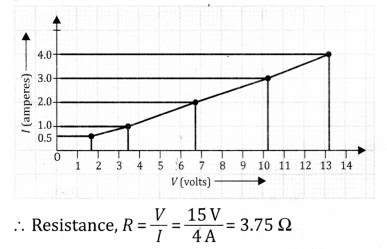Question 8.
When a 12 V battery is connected across an unknown resistor, there is a current of 2.5 mA in the circuit. Find the value of the resistance of the resistor.
Given: V= 12 volts, I = 2.5 mA = 2.5 x 10 -3 A
Resistance, R = ?
∴ Resistance =  V I  =  12 2.5 × 10 3  = 4.8 x 10 3 Ω

Question 9.
A battery of 9 V is connected in series with resistors of 0.2 Ω, 0.3 Ω, 0.4 Ω, 0.5 Ω and 12 Ω, respectively. How much current would flow through the 12 Ω resistor?
Given: V= 9 volts
Applying the formula R = R 1 + R 2+ R 3+ R 4 + R s, we get
R = 0.2 + 0.3 + 0.4 + 0.5 + 12 = 13.4 Ω
By Ohm’s law, we have
V=RI or
or 9 = 13.4 x I
or  I = 9 13.4 = 0.67 A
Hence, the current flows through the 12 Ω resistor is 0.67 A.

Question 10.
How many 176 Ω resistors (in parallel) are required to carry 5 A on a 220 V line?
Given: V= 220 volts, I= 5 A
Resistance of each resistor, R = 176 Ω
Number of resistors = n, We know that V = R pIQuestion 11.
Show how you would connect three resistors each of resistance 6 ft, so that the combination has a resistance of
(a) 9 Ω, and
(b) 4 Ω. ,
(a) In order to get a resistance of 9 ft from the given three resistors, two resistors can be connected in parallel and this parallel combination in series with the third one.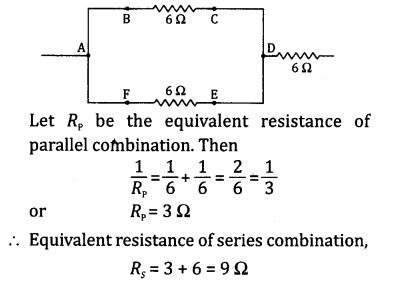(b) In order to get a resistance of 4 ft from the given three resistors, two resistors can be connected in series and this series in parallel with the third one.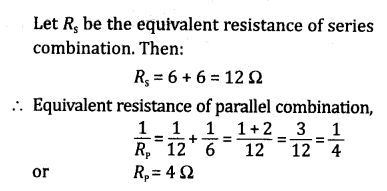Question 12.
Several electric bulbs designed to be used on a 220 V electric supply line, are rated 10 W. How many lamps can be connected in parallel with each other across the two wires of 220 V line, if the maximum allowable current is 5 A?
Given: Potential, V = 220 volts, P = 10 W, I = 5 A
Let number of bulbs = n
Applying the formula P = VI, we get: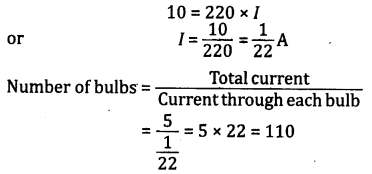Hence, 110 bulbs can be connected is parallel.

Question 13.
A hot plate of an electric oven connected to a 220 volt line has two resistance coils A and B, each of 24 Ω resistance, which may be used separately, in series, or in parallel. What are the currents in the three cases?
Given: Voltage, V = 220 V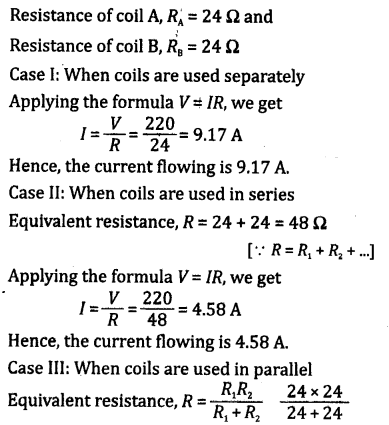Question 14.
Compare the power used in the 2 Ω resistor in each of the following circuits:
(a) A 6 V battery in series with 1 Ω and 2 Ω resistors, and
(b) a 4 V battery in parallel with 12 Ω and 2 Ω resistors.
(a) Given: Voltage, V= 6 V
Equivalent resistance, R = 1 + 2 = 3 Ω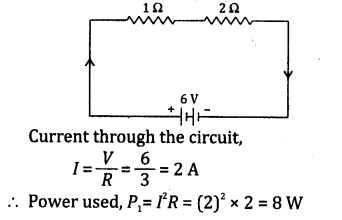(b) Since the battery is in parallel with 12 Ω and 2 Ω resistors
∴ Potential difference across 2 Ω resistor,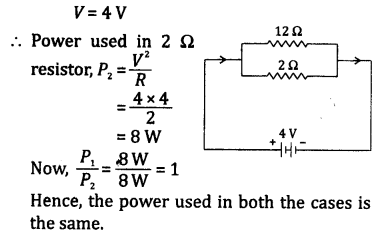Question 15.
Two lamps, one rated 100 W at 220 V, and the other 60 W at 220 V, are connected in parallel to electric mains supply. What current is drawn from the line if the supply voltage is 220 V?
Given: P 1 = 100 W, P 2 = 60 W and 7= 220 V
Resistance of first lamp,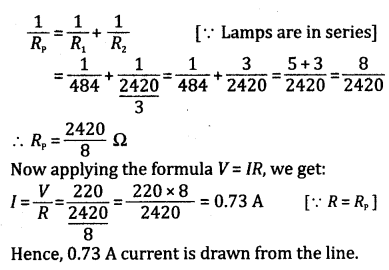Question 16.
Which uses more energy, a 250 W TV set in 1 hr or a 1200 W toaster in 10 minutes?
Energy used by TV set = Power x Time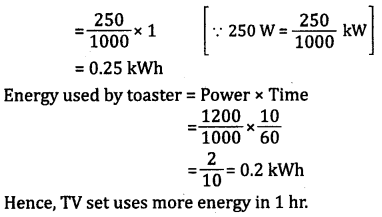Question 17.
An electric heater of resistance 8 W draws a current of 15 A from the service mains for 2 hr. Calculate the rate at which heat is developed in the heater.
Given: Resistance, R = 8 Ω, t = 2 hr, I = 15 A
Rate of heat developed,  H t = I 2 R T t
Power = I 2R = (15) 2 x 8 = 1800 W

Question 18.
Explain the following:,
(a) Why is the tungsten used almost exclusively for filament of electric lamps?
(b) Why are the conductors of electric heating devices such as bread toaster and electric irons, made of an alloy rather than a pure metal?
(c) Why is the series arrangement not used for domestic circuits?
(d) How does the resistance of a wire vary with its area of cross-section?
(e) Why are copper and aluminium wires usually employed for electricity transmission?